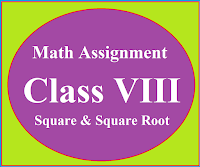## Posts

Showing posts from April, 2019

### Math Assignment Class VIII | Square & Square RootMath Assignment  Class VIII | Square & Square Root Download or Print free  assignment with answer key  for   Class  8 Squares and  Square Roots.   Important and extra questions that cover all topics of square and square root and is useful and helpful for the students. Math Assignment  Class VIII | Square & Square Root LEVEL -1

### Polynomials basic concepts-cbse mathematicsPolynomial Chapter 2 Different types of polynomials, degree of polynomials, relationship between zeroes and coefficients of quadratic and cubic polynomials, pair of linear equations in two variables, methods of solving pair of linear equations in two variables. COMMON TOPICS OF IX & X STANDARD